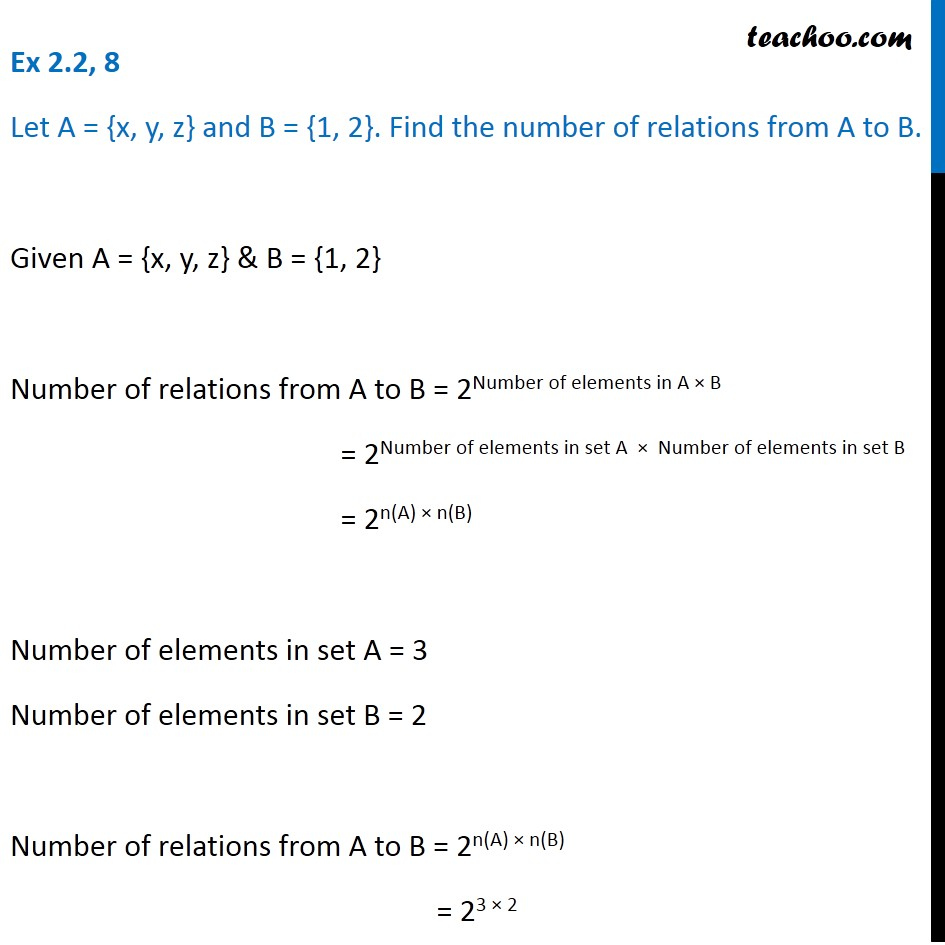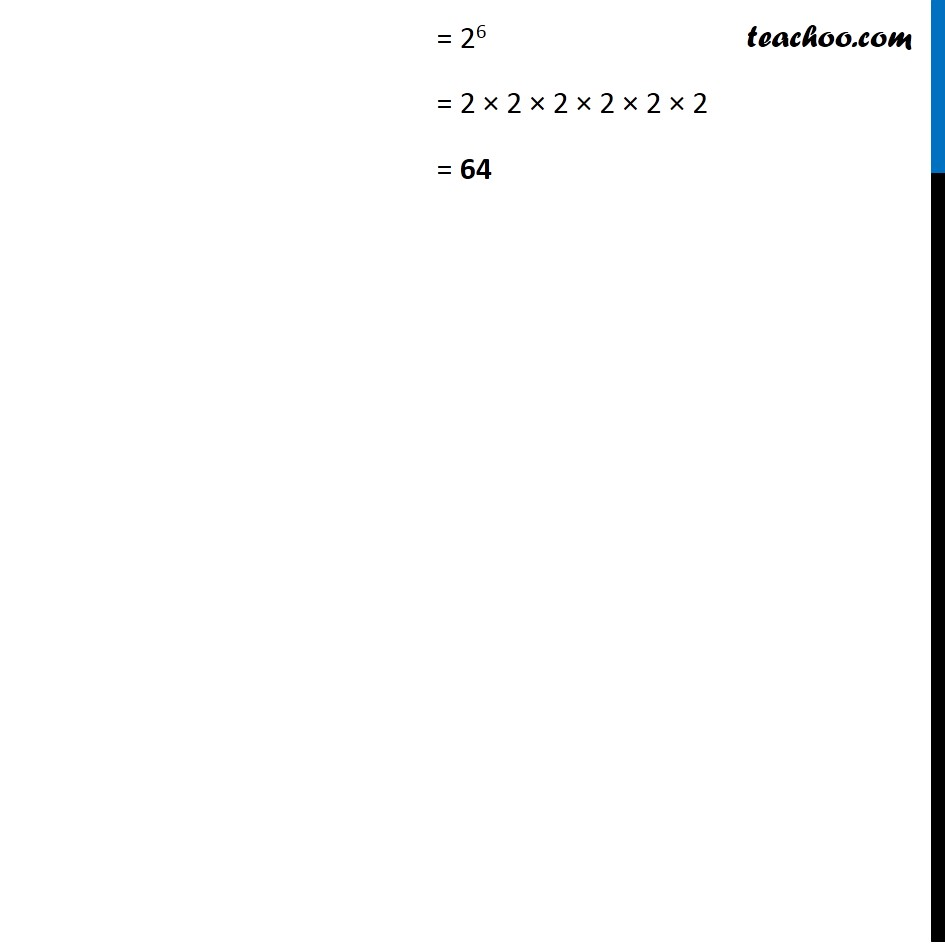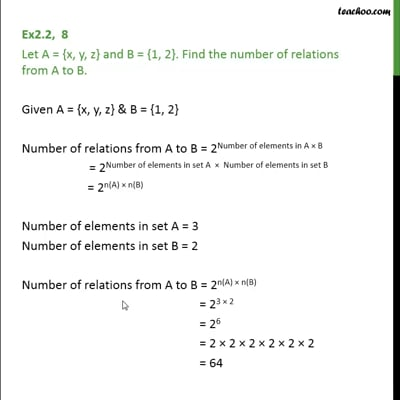Ex 2.2

Chapter 2 Class 11 Relations and Functions
Serial order wiseThis video is only available for Teachoo black users

Solve all your doubts with Teachoo Black (new monthly pack available now!)

### Transcript

Ex 2.2, 8 Let A = {x, y, z} and B = {1, 2}. Find the number of relations from A to B. Given A = {x, y, z} & B = {1, 2} Number of relations from A to B = 2Number of elements in A × B = 2Number of elements in set A × Number of elements in set B = 2n(A) × n(B) Number of elements in set A = 3 Number of elements in set B = 2 Number of relations from A to B = 2n(A) × n(B) = 23 × 2 = 26 = 2 × 2 × 2 × 2 × 2 × 2 = 64